Latest Banking jobs   »

# Reasoning Ability Quiz For LIC ADO Mains 2023-21st March

Directions (1-5): A number arrangement machine when given an input line of numbers rearranges them following a particular rule in each step. The given is an illustration of input and rearrangement.

Input: 684 957 735 407 482 548 747 277
Step I: 36 63 45 33 28 51 36 32
Step II: 648 894 690 374 454 497 711 245
Step III: 244 373 453 496 647 689 710 893
Step IV: 10 13 12 19 17 23 8 20
Step V: 20 12 10 8 23 19 17 13

As per the rules followed in the steps given above, find out in each of the given questions the appropriate step for the input given.

Input: 456 728 731 842 994 986 642 814

Q1. Which of the following number is 4th from the left side in step IV?
(a) 19
(b) 16
(c) 22
(d) 12
(e) None of these

Q2. Which of the following number is 6th from the right side in step II?
(a) 698
(b) 814
(c) 950
(d) 917
(e) None of these

Q3. What is the sum of the numbers which is 3rd from the right end in step III and 4th number from the left end in step IV?
(a) 864
(b) 893
(c) 835
(d) 992
(e) None of these

Q4. What is the product of number which is 2nd from the left end in step IV and the number which is 3rd from the right end of step V?
(a) 168
(b) 144
(c) 132
(d) 165
(e) None of these

Q5. Which of following step is the final step?
(a) 22 22 18 16 14 12 19 5
(b) 22 18 22 16 12 14 19 5
(c) 22 22 18 14 16 19 12 5
(d) 22 22 16 14 18 12 19 5
(e) None of these

Directions (6-7): Answer the questions based on the information given below.
If X % Y means X is mother of Y,
X\$Y means X is father of Y,
X# Y means X is sister of Y,
X*Y means X is brother of Y,
X+Y means X is wife of Y,
X@Y means X is husband of Y

Q6. If ‘P%S\$V#T@U%M’ is true then how is V related to M?
(a) Father
(b) Brother
(c) Aunt
(d) Grandfather
(e) Grandmother

Q7. If ‘Z\$L*X+S\$Q#B’ is true then how is B related to S?
(a) Father
(b) Sister
(c) Daughter
(d) Son
(e) Cannot be determined

Directions (8-9): Answer the questions based on the information given below.
If, P@Q means P is the mother of Q,
P% Q means P is the sister of Q,
P\$Q means P is the father of Q,
P #Q means P is the brother of Q,
P&Q means P is the daughter of Q.

Q8. In expression ‘P%Q\$R#S@T&U #V’ how is R related to T?
(a) Uncle
(b) Aunt
(c) Mother
(d) Father
(e) None of the above

Q9. In expression ‘A&B\$C&D%E%F&G’ how is G related to A?
(a) Paternal Grandfather
(b) Maternal Grandfather
(c) Maternal Grandmother
(d) Either (b) or (c)
(e) None of the above

Directions (10-12): Answer the questions based on the information given below.
S × T means S is the wife of T,
S – T means S is the brother of T,
S + T means S is the sister of T,
S ÷ T means S is the father of T.

Q10. How is W related to H in the expression ‘T÷H×K–L÷W’?
(a) Son
(b) Nephew
(c) Niece
(d) Son- in -law
(e) None of the above

Q11. Which of the following statement is true if the expression is ‘D+R×T–Y÷U’ true?
(a) U is niece of T.
(b) D is brother-in-law of T
(c) R is sister-in-law of Y
(d) T is father of U
(e) All are correct

Q12. How many female members are in the family if the expression ‘J+K×Y÷R+C–X+S×W’ is true?
(a) One
(b) Two
(c) Five
(d) Four
(e) Can’t be determined

Directions (13-15): Answer the questions based on the information given below.
D @ E – D is the mother of E
D & E – D is the sister of E
D % E – D is the father of E
D # E – D is the brother of E
D ¥ E – D is the wife of E
D £ E – D is the son of E
D ! E – D is daughter of E

Q13. If ‘W % S @ T £ Z % X ¥ H’, then how X is related to W?
(a) Granddaughter
(b) Daughter
(c) Son
(d) Sister-in-law
(e) None of the above

Q14. If ‘H#K&T¥S%C@V, B£K!X’ then who among the following is son-in-law of X?
(a) B
(b) C
(c) H
(d) S
(e) None of the above

Q15. If ‘E!R&V#G£A%Q@P¥J’, then which of the following statement is not true?
(a) V is uncle of P.
(b) R is daughter of A
(c) E is nephew of G.
(d) J is son-in-law of Q.
(e) Q is sister of V.

Solutions

Solution (1-5):
Sol. Different logic is applied in each step.
Step I: Number in step I are arranged based on certain conditions.
1. If the sum of the digits of a number is an even number, then multiply the sum by 2.
2. If the sum of the digits of a number is an odd number, then multiply the sum by 3.
Step II: Now, subtract each number of step I from the number given in input such as the first number of step I from the left side is subtracted from number of the input from the left side and so on…
Step Ill: Arrange the number given in step II, in ascending order from left to right after subtracting 1 from each of the numbers.
Step IV: The sum of digits of the number in step III is written from left to right.
Step V: First arrange all the even numbers in descending order then arrange all the odd numbers in descending order.

Given input: 456 728 731 842 994 986 642 814
Step I: 45 51 33 28 44 69 24 39
Step II: 411 677 698 814 950 917 618 775
Step III: 410 617 676 697 774 813 916 949
Step IV: 5 14 19 22 18 12 16 22
Step V: 22 22 18 16 14 12 19 5

S1. Ans. (c)
S2. Ans. (a)
S3. Ans. (c)
S4. Ans. (a)
S5. Ans. (a)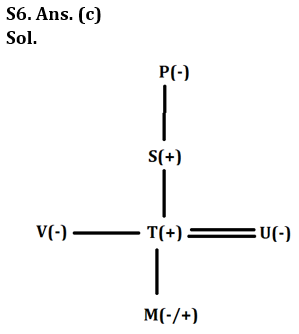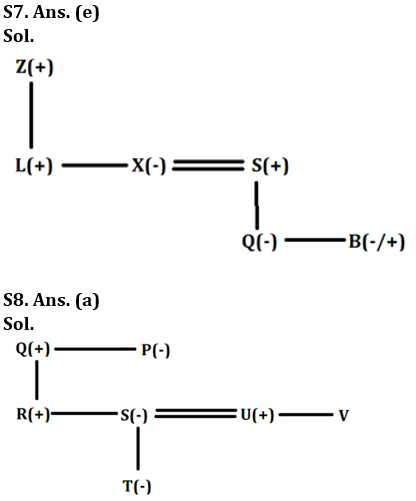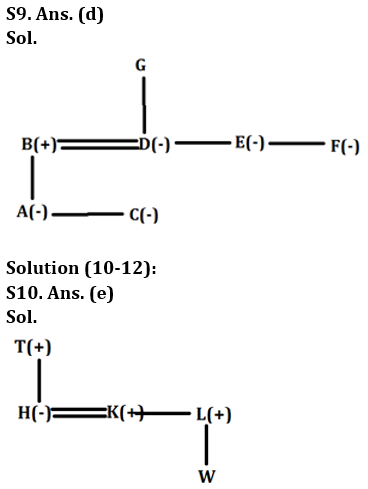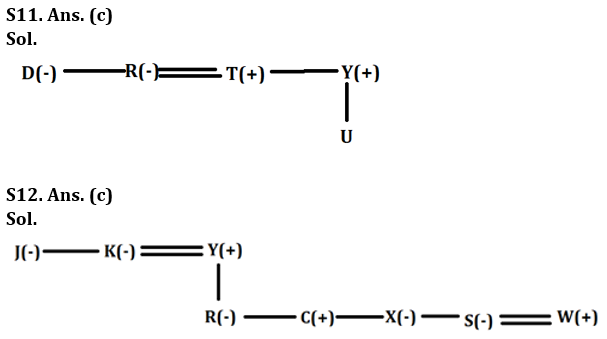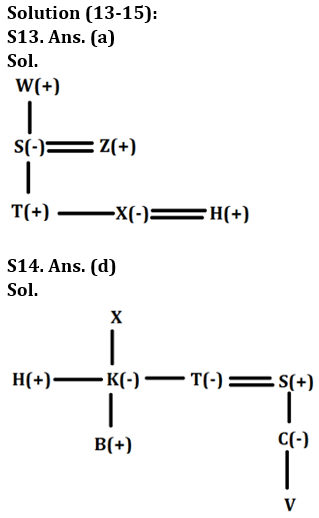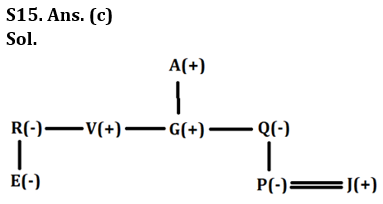## FAQs

### When is the LIC ADO Mains Exam scheduled?

The LIC ADO Mains exam is scheduled to be held on 23 April 2023

#### Congratulations!Union Budget 2023-24: Free PDF